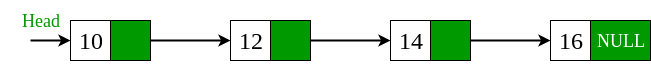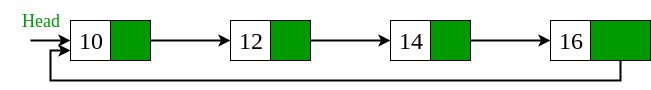GeeksforGeeks App
Open AppBrowser
Continue

Given a singly linked list, we have to convert it into circular linked list. For example, we have been given a singly linked list with four nodes and we want to convert this singly linked list into circular linked list.Approach: The idea is to traverse the singly linked list and check if the node is the last node or not. If the node is the last node i.e pointing to NULL then make it point to the starting node i.e head node. Below is the implementation of this approach.

### Algorithm:

```Here are the algorithmic steps to convert a singly linked list into a circular linked list:

Step 1 : Initialize a pointer current to the head node of the singly linked list.

Step 2 : Traverse the linked list by moving the current pointer to the next node until it
reaches the last node (i.e., the node whose next pointer is NULL).

Step 3 : Set the next pointer of the last node to point back to the head node

Note that step 3 is what actually converts the singly linked list into a

Implementation:

## C++

 `// Program for converting singly linked list``// into circular linked list.``#include``#include ``using` `namespace` `std;` `/* Linked list node */``struct` `Node {``    ``int` `data;``    ``struct` `Node* next;``};` `// Function that convert singly linked list``// into circular linked list.``struct` `Node* circular(``struct` `Node* head)``{``    ``// declare a node variable start and``    ``// assign head node into start node.``    ``struct` `Node* start = head;` `    ``// check that while head->next not equal``    ``// to NULL then head points to next node.``    ``while` `(head->next != NULL)``        ``head = head->next;``        ` `    ``// if head->next points to NULL then``    ``// start assign to the head->next node.``    ``head->next = start;``    ``return` `start;``}` `void` `push(``struct` `Node** head, ``int` `data)``{``    ``// Allocate dynamic memory for newNode.``    ``struct` `Node* newNode = (``struct` `Node*)``malloc``                          ``(``sizeof``(``struct` `Node));` `    ``// Assign the data into newNode.``    ``newNode->data = data;` `    ``// newNode->next assign the address of``    ``// head node.``    ``newNode->next = (*head);` `    ``// newNode become the headNode.``    ``(*head) = newNode;``}` `// Function that display the elements of``// circular linked list.``void` `displayList(``struct` `Node* node)``{``    ``struct` `Node* start = node;` `    ``while` `(node->next != start) {``        ``cout << ``" "` `<< node->data;``        ``node = node->next;``    ``}``    ` `    ``// Display the last node of circular``    ``// linked list.``    ``cout << ``" "` `<< node->data;``}` `// Driver program to test the functions``int` `main()``{``    ``// Start with empty list``    ``struct` `Node* head = NULL;` `    ``// Using push() function to construct``    ``// singly linked list``    ``// 17->22->13->14->15``    ``push(&head, 15);``    ``push(&head, 14);``    ``push(&head, 13);``    ``push(&head, 22);``    ``push(&head, 17);` `    ``// Call the circular_list function that``    ``// convert singly linked list to circular``    ``// linked list.``    ``circular(head);``    ``cout << ``"Display list: \n"``;``    ``displayList(head);` `    ``return` `0;``}`

## C

 `//importing basic libraries for c program``#include ``#include `` ` `//Now define structure of linked list``struct` `Node {``    ``int` `data;``    ``struct` `Node* next;``};` `//function to convert linear linked list into circular linked list``struct` `Node* circular(``struct` `Node* head){``      ``//declaring a new node name start and assigning head to it.``      ``struct` `Node* start=head;``      ` `       ``//now find the last element of the linked list using while loop``       ``while``(head->next != NULL){``        ``head = head->next;``       ``}``       ` `       ``//now assign start i.e head as next to last elementny head->next=start``       ``head->next = start;``       ``return` `start;``}` `void` `push(``struct` `Node** head, ``int` `data)``{``    ``// Allocate dynamic memory for newNode.``    ``struct` `Node* newNode = (``struct` `Node*)``malloc``(``sizeof``(``struct` `Node));` `    ``//Assign the data into newNode.``    ``newNode->data = data;``     ` `    ``// head node.``    ``newNode->next = (*head);` `    ``// newNode become the headNode.``    ``(*head) = newNode;``}` `// Function that display the elements of circular linked list.``void` `displayList(``struct` `Node* node)``{``    ``struct` `Node* start = node;` `    ``while` `(node->next != start) {``        ``printf``(``"%d "``, node->data);``        ``node = node->next;``    ``}``    ` `    ` `    ``printf``(``"%d "``, node->data);``}` `// Driver program to test the functions``int` `main()``{``     ``// Start with empty list``    ``struct` `Node* head = NULL;` `    ``// Using push() function to construct``    ``// singly linked list``    ``// 17->22->13->14->15``    ` `    ``push(&head, 15);``    ``push(&head, 14);``    ``push(&head, 13);``    ``push(&head, 22);``    ``push(&head, 17);` `    ``// Call the circular_list function that``    ``// convert singly linked list to circular``    ``// linked list.``    ``circular(head);` `    ``printf``(``"Display list: \n"``);``    ``displayList(head);` `    ``return` `0;``}`

## Java

 `// Program for converting``// singly linked list into``// circular linked list.``import` `java.util.*;``import` `java.io.*;` `public` `class` `GFG``{` `/*Linked list node */``static` `class` `Node``{``    ``int` `data;``    ``Node next;``};` `// Function that convert``// singly linked list into``// circular linked list.``static` `Node circular(Node head)``{``    ``// declare a node variable``    ``// start and assign head``    ``// node into start node.``    ``Node start = head;` `    ``// check that while head.next``    ``// not equal to null then head``    ``// points to next node.``    ``while` `(head.next != ``null``)``        ``head = head.next;``        ` `    ``// if head.next points to null``    ``// then start assign to the``    ``// head.next node.``    ``head.next = start;``    ``return` `start;``}` `static` `Node push(Node head, ``int` `data)``{``    ``// Allocate dynamic memory``    ``// for newNode.``    ``Node newNode = ``new` `Node();` `    ``// Assign the data into newNode.``    ``newNode.data = data;` `    ``// newNode.next assign the``    ``// address of head node.``    ``newNode.next = (head);` `    ``// newNode become the headNode.``    ``(head) = newNode;``    ` `    ``return` `head;``}` `// Function that display the elements``// of circular linked list.``static` `void` `displayList( Node node)``{``    ``Node start = node;` `    ``while` `(node.next != start)``    ``{``        ``System.out.print(``" "``+ node.data);``        ``node = node.next;``    ``}``    ` `    ``// Display the last node of ``    ``// circular linked list.``    ``System.out.print(``" "` `+ node.data);``}` `// Driver Code``public` `static` `void` `main(String args[])``{``    ``// Start with empty list``    ``Node head = ``null``;` `    ``// Using push() function to``    ``// convert singly linked list``    ``// 17.22.13.14.15``    ``head = push(head, ``15``);``    ``head = push(head, ``14``);``    ``head = push(head, ``13``);``    ``head = push(head, ``22``);``    ``head = push(head, ``17``);` `    ``// Call the circular_list function``    ``// that convert singly linked``    ``// list to circular linked list.``    ``circular(head);` `    ``System.out.print(``"Display list: \n"``);``    ``displayList(head);``}``}` `// This code is contributed``// by Arnab Kundu`

## Python3

 `# Python3 program for converting``# singly linked list into``# circular linked list.``import` `sys` `# Linked list node``class` `Node:``    ``def` `__init__(``self``,data):``        ``self``.data ``=` `data``        ``self``.``next` `=` `None` `def` `push(head, data):``    ``if` `not` `head:``        ``return` `Node(data)` `    ``# Allocate dynamic memory``    ``# for newNode.``    ``# Assign the data into newNode.``    ``newNode ``=` `Node(data)` `    ``# newNode.next assign the``    ``# address of head node.``    ``newNode.``next` `=` `head` `    ``# newNode become the headNode.``    ``head ``=` `newNode``    ``return` `head` `# Function that convert``# singly linked list into``# circular linked list.``def` `circular(head):` `    ``# declare a node variable``    ``# start and assign head``    ``# node into start node.``    ``start ``=` `head` `    ``# check that while head.next``    ``# not equal to null then head``    ``# points to next node.``    ``while``(head.``next` `is` `not` `None``):``        ``head ``=` `head.``next` `    ``# if head.next points to null``    ``# then start assign to the``    ``# head.next node.    ``    ``head.``next` `=` `start``    ``return` `start` `# Function that display the elements``# of circular linked list.``def` `displayList(node):``    ``start ``=` `node``    ``while``(node.``next` `is` `not` `start):``        ``print``(``"{} "``.``format``(node.data),end``=``"")``        ``node``=``node.``next` `    ``# Display the last node of``    ``# circular linked list.``    ``print``(``"{} "``.``format``(node.data),end``=``"")` `# Driver Code``if` `__name__``=``=``'__main__'``:``    ` `    ``# Start with empty list``    ``head``=``None` `    ``# Using push() function to``    ``# convert singly linked list``    ``# 17.22.13.14.15``    ``head``=``push(head,``15``)``    ``head``=``push(head,``14``)``    ``head``=``push(head,``13``)``    ``head``=``push(head,``22``)``    ``head``=``push(head,``17``)` `    ``# Call the circular_list function``    ``# that convert singly linked``    ``# list to circular linked list.``    ``circular(head)``    ``print``(``"Display List:"``)``    ``displayList(head)` `# This Code is Contributed By Vikash Kumar 37`

## C#

 `// C# Program for converting``// singly linked list into``// circular linked list.``using` `System;` `class` `GFG``{` `    ``/*Linked list node */``    ``public` `class` `Node``    ``{``        ``public` `int` `data;``        ``public` `Node next;``    ``};` `    ``// Function that convert``    ``// singly linked list into``    ``// circular linked list.``    ``static` `Node circular(Node head)``    ``{``        ``// declare a node variable``        ``// start and assign head``        ``// node into start node.``        ``Node start = head;` `        ``// check that while head.next``        ``// not equal to null then head``        ``// points to next node.``        ``while` `(head.next != ``null``)``            ``head = head.next;` `        ``// if head.next points to null``        ``// then start assign to the``        ``// head.next node.``        ``head.next = start;``        ``return` `start;``    ``}` `    ``static` `Node push(Node head, ``int` `data)``    ``{``        ``// Allocate dynamic memory``        ``// for newNode.``        ``Node newNode = ``new` `Node();` `        ``// Assign the data into newNode.``        ``newNode.data = data;` `        ``// newNode.next assign the``        ``// address of head node.``        ``newNode.next = (head);` `        ``// newNode become the headNode.``        ``(head) = newNode;` `        ``return` `head;``    ``}` `    ``// Function that display the elements``    ``// of circular linked list.``    ``static` `void` `displayList( Node node)``    ``{``        ``Node start = node;` `        ``while` `(node.next != start)``        ``{``            ``Console.Write(``" "` `+ node.data);``            ``node = node.next;``        ``}` `        ``// Display the last node of``        ``// circular linked list.``        ``Console.Write(``" "` `+ node.data);``    ``}` `    ``// Driver Code``    ``public` `static` `void` `Main(String []args)``    ``{``        ``// Start with empty list``        ``Node head = ``null``;` `        ``// Using push() function to``        ``// convert singly linked list``        ``// 17.22.13.14.15``        ``head = push(head, 15);``        ``head = push(head, 14);``        ``head = push(head, 13);``        ``head = push(head, 22);``        ``head = push(head, 17);` `        ``// Call the circular_list function``        ``// that convert singly linked``        ``// list to circular linked list.``        ``circular(head);` `        ``Console.Write(``"Display list: \n"``);``        ``displayList(head);``    ``}``}` `// This code is contributed 29AjayKumar`

## Javascript

 ``

Output

```Display list:
17 22 13 14 15```

Time Complexity: O(n), As we need to move through the whole list to get hold of the last node.
Auxiliary Space: O(1), As constant extra space is used.

### Example:

Here’s a C++ implementation of a function that converts a singly linked list into a circular linked list with minimum time complexity:

## C++

 `#include ``using` `namespace` `std;` `struct` `Node {``    ``int` `data;``    ``Node* next;``    ``Node(``int` `value) {``        ``data = value;``        ``next = NULL;``    ``}``};` `void` `convertToCircular(Node* head) {``    ``Node* current = head;``    ``while` `(current->next != NULL) {``        ``current = current->next;``    ``}``    ``current->next = head;``}` `void` `printList(Node* head) {``    ``Node* current = head;``    ``do` `{``        ``cout << current->data << ``" "``;``        ``current = current->next;``    ``} ``while` `(current != head);``}` `int` `main() {``    ``Node* head = ``new` `Node(1);``    ``head->next = ``new` `Node(2);``    ``head->next->next = ``new` `Node(3);``    ``head->next->next->next = ``new` `Node(4);` `    ``convertToCircular(head);` `    ``cout << ``"The circular linked list is: "``;``    ``printList(head);` `    ``return` `0;``}`

## Java

 `import` `java.io.*;` `public` `class` `Node {``    ``// Define a class Node``    ``public` `static` `class` `NodeClass {``        ``int` `data;``        ``NodeClass next;``        ``// Constructor for Node class``        ``public` `NodeClass(``int` `value)``        ``{``            ``data = value;``            ``next = ``null``;``        ``}``    ``}` `    ``// Define a method to convert a linked list to a``    ``// circular linked list``    ``public` `static` `void` `convertToCircular(NodeClass head)``    ``{``        ``NodeClass current = head;``        ``while` `(current.next != ``null``) {``            ``current = current.next;``        ``}``        ``current.next = head;``    ``}` `    ``// Define a method to print the circular linked list``    ``public` `static` `void` `printList(NodeClass head)``    ``{``        ``NodeClass current = head;``        ``do` `{``            ``System.out.print(current.data + ``" "``);``            ``current = current.next;``        ``} ``while` `(current != head);``    ``}` `    ``// Main method``    ``public` `static` `void` `main(String[] args)``    ``{``        ``// Create a new linked list``        ``NodeClass head = ``new` `NodeClass(``1``);``        ``head.next = ``new` `NodeClass(``2``);``        ``head.next.next = ``new` `NodeClass(``3``);``        ``head.next.next.next = ``new` `NodeClass(``4``);` `        ``// Convert the linked list to a circular linked list``        ``convertToCircular(head);` `        ``// Print the circular linked list``        ``System.out.println(``"The circular linked list is: "``);``        ``printList(head);``    ``}``}`

## Python3

 `# Define a class Node``class` `Node:``    ``def` `__init__(``self``, value):``        ``self``.data ``=` `value``        ``self``.``next` `=` `None` `# Define a method to convert a linked list to a``# circular linked list`  `def` `convertToCircular(head):``    ``current ``=` `head``    ``while` `current.``next` `!``=` `None``:``        ``current ``=` `current.``next``    ``current.``next` `=` `head` `# Define a method to print the circular linked list`  `def` `printList(head):``    ``current ``=` `head``    ``while` `True``:``        ``print``(current.data, end``=``" "``)``        ``current ``=` `current.``next``        ``if` `current ``=``=` `head:``            ``break`  `# Main method``if` `__name__ ``=``=` `"__main__"``:``    ``# Create a new linked list``    ``head ``=` `Node(``1``)``    ``head.``next` `=` `Node(``2``)``    ``head.``next``.``next` `=` `Node(``3``)``    ``head.``next``.``next``.``next` `=` `Node(``4``)` `    ``# Convert the linked list to a circular linked list``    ``convertToCircular(head)` `    ``# Print the circular linked list``    ``print``(``"The circular linked list is:"``)``    ``printList(head)`

## C#

 `using` `System;` `public` `class` `Node {``    ``public` `int` `data;``    ``public` `Node next;``    ``public` `Node(``int` `value) {``        ``data = value;``        ``next = ``null``;``    ``}``}` `public` `class` `LinkedList {``    ``public` `static` `void` `ConvertToCircular(Node head) {``        ``Node current = head;``        ``while` `(current.next != ``null``) {``            ``current = current.next;``        ``}``        ``current.next = head;``    ``}` `    ``public` `static` `void` `PrintList(Node head) {``        ``Node current = head;``        ``do` `{``            ``Console.Write(current.data + ``" "``);``            ``current = current.next;``        ``} ``while` `(current != head);``    ``}``}` `class` `Program {``    ``static` `void` `Main(``string``[] args) {``        ``Node head = ``new` `Node(1);``        ``head.next = ``new` `Node(2);``        ``head.next.next = ``new` `Node(3);``        ``head.next.next.next = ``new` `Node(4);` `        ``LinkedList.ConvertToCircular(head);` `        ``Console.Write(``"The circular linked list is: "``);``        ``LinkedList.PrintList(head);``    ``}``}`

## Javascript

 `class Node {``  ``constructor(value) {``    ``this``.data = value;``    ``this``.next = ``null``;``  ``}``}` `function` `convertToCircular(head) {``  ``let current = head;``  ``while` `(current.next !== ``null``) {``    ``current = current.next;``  ``}``  ``current.next = head;``}` `function` `printList(head) {``  ``let current = head;``  ``do` `{``    ``console.log(current.data + ``" "``);``    ``current = current.next;``  ``} ``while` `(current !== head);``}` `let head = ``new` `Node(1);``head.next = ``new` `Node(2);``head.next.next = ``new` `Node(3);``head.next.next.next = ``new` `Node(4);` `convertToCircular(head);` `console.log(``"The circular linked list is: "``);``printList(head);`

Output

`The circular linked list is: 1 2 3 4 `

Time Complexity: O(n), It is O(n) because we are traversing the whole the linked list so it requires n iteration.
Auxiliary Space: O(1),  as here only constant space is used but if for general linked list it is O(n). Hence it is O(1).

### Explanation:

In this implementation, the convertToCircular() function takes a pointer to the head node of a singly linked list as input and modifies the last node of the linked list to point back to the head node, effectively converting the singly linked list into a circular linked list. The function does this by initializing a current pointer to the head node, and then traversing the linked list until it reaches the last node. It then sets the next pointer of the last node to the head node, creating a circular link.

The printList() function is used to print the values of the nodes in the circular linked list. It does this by initializing a current pointer to the head node, and then traversing the linked list using a do-while loop that continues until the current pointer reaches the head node again. This ensures that all nodes in the circular linked list are printed.

The main() function creates a sample singly linked list and calls the convertToCircular() function to convert it to a circular linked list. It then calls the printList() function to print the values of the nodes in the circular linked list.

This article is contributed by Dharmendra kumar. If you like GeeksforGeeks and would like to contribute, you can also write an article using write.geeksforgeeks.org or mail your article to review-team@geeksforgeeks.org. See your article appearing on the GeeksforGeeks main page and help other Geeks.

My Personal Notes arrow_drop_up